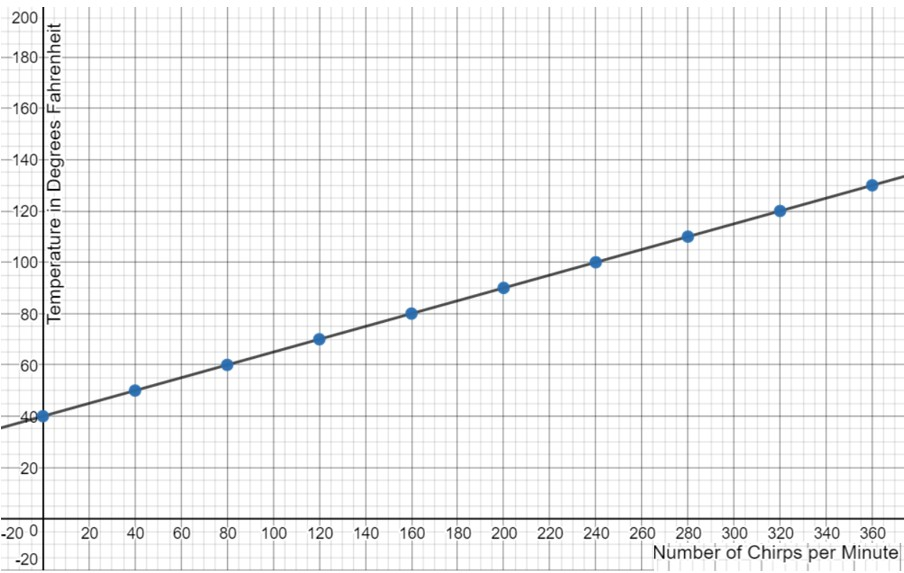# Equations of Linear Situations

In the following exercises, you will use multiple representations to write linear equations.

## A Linear Situation

When Henry started climbing Hogsback (a local mountain), he was at an elevation of 4,500 feet. He climbed at a rate of 40 feet per hour.

1.  Write an equation to determine Henry’s elevation over time, as he climbed the mountain.  Let $$e$$ represent Henry’s elevation and $$t$$ represent time in hours.

2.  Use your equation to determine Henry’s elevation after 8 hours of climbing.

3.  Use your equation to determine the number of hours that Henry must climb to reach 5,020 feet.

## A Table

A storm front moved into the local area early this morning.  The table below records the temperature, in degrees, during the first four hours of the storm.  Assume that the storm continues for several days and that the temperature changes in the same manner over the next 8 hours.

 Time (in hours) Temperature (in degrees Fahrenheit) 0 $$67$$ 1 $$63$$ 2 $$59$$ 3 $$55$$ 4 $$51$$

1.  Write an equation to determine the temperature during the first eight hours of the storm.  (Hint: first define two variables).

2.  Use your equation to determine the temperature at $$2.5$$ hours since the storm front moved in.

3.  When will the temperature reach $$37^{\circ}$$?

## A Graph

Temperature can be estimated based on the number of chirps a cricket makes per minute.  Crickets will chirp when temperatures reach at least $$40^{\circ}$$ Fahrenheit.  The graph below represents the estimated temperature based on the number of chirps per minute. Graph is created using Desmos.com.1.  Write an equation to find the approximate temperature based on the number of chirps counted per minute. (Hint: First define two variables.)

2.  Use your equation to find the temperature when 300 chirps per minute are counted.

3.  Use your equation to estimate the number of chirps per minute that should be counted if the temperature is $$62^{\circ}$$.